Home > CC4 > Chapter A > Lesson A.1.9 > ProblemA-96

A-96.

Solve each equation. Record your work and check your answer. Homework Help ✎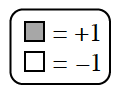1.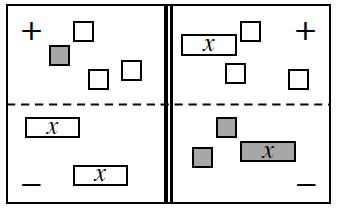2.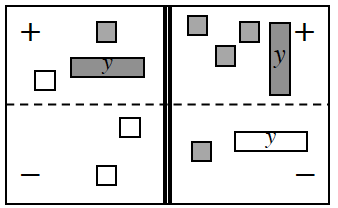3.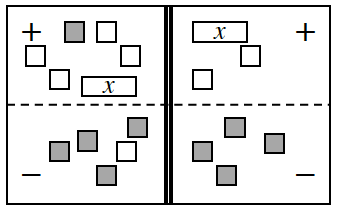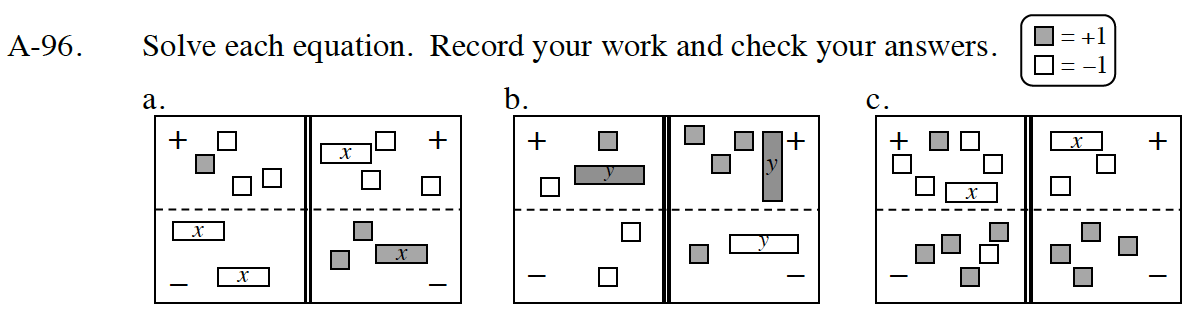The expression for the left side is 1 − 3 − (2x). The expression for the right side is −x − 3 −(2 + x). Simplify each side by flipping tiles from the negative to the positive region and removing zero pairs. Record your work.

2x − 2 = −2x − 5

4x = −3

$x\ =\ -\frac{3}{4}$

The simplified expression for the left side is y + 2, for the right side is 2y + 2

Be sure to record your work and check your answer.

See parts (a) and (b).